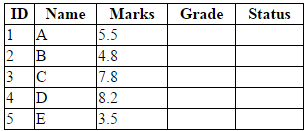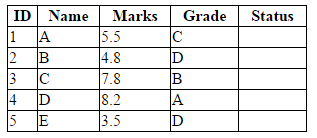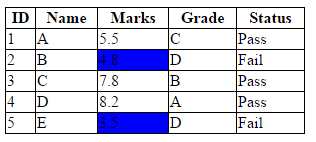# The HTML DOM and Traversing the DOM by eq() and find() Methods in jQuery

### In this post, I want to introduce the HTML DOM and explain how to traverse the DOM by eq() and find() mehods in jQuery.

· Web Dev Zone · Tutorial
Save
20.36K Views

## Introduction

jQuery is a JavaScript library. The purpose of jQuery is to make it much easier to use JavaScript on your website.

In this post, I want to introduce the HTML DOM and explain how to traverse the DOM by eq() and find() methods in jQuery.

### Prerequisite

• Download the jQuery library from the website jquery.com and save your downloaded jQuery file as jquery.js in a new folder on your machine. You're also going to add an HTML file to this folder, so create a mypage.html page, too. Finally, you'll want to write all the JavaScript in an individual file, so create myjquery.js.

• Include jQuery library (jquery.js) and JS file (myjquery.js) in mypage.html (using <script> tag):

``````<!DOCTYPE html>

<html>
<title>My webpage</title>
<script src="jquery.js"></script>
<script src="myjquery.js"></script>
<body>
<!--…webpage contents here-->
</body>
</html>

``````
• In myjquery.js file, all my jquery code are put inside a document ready event

``````\$(document).ready(function(){

// my code here
});``````

This is to prevent any jQuery code from running before the document has finished loading (is ready).

### jQuery Selectors

jQuery selectors allow you to select and manipulate HTML elements. They are used to select HTML elements based on their name, id, classes, etc. All selectors in jQuery start with the dollar sign and parentheses: \$().

Example: select all p elements on a page like this: \$('p'); find an element with a specific id attribute like this: \$("#idname"); find an element with a specific class attribute like this: \$(".classname").

### The HTML DOM (Document Object Model)

When a web page is loaded, the browser creates a Document Object Model (DOM) of the page.

The DOM is a platform and language-neutral interface that allows programs and scripts to dynamically access and update the content, structure, and style of a document. (W3C)

The HTML DOM is a standard for how to get, change, add, or delete HTML elements.

### Traversing the DOM

Traversing, which means "move through", is used to select (or find) HTML elements based on their relation to other elements (such as ancestors, descendants, siblings… in a family tree).

Consider the following HTML fragment:

``````<div>
<ul>
<li>
<span> item 1 </span>
</li>
<li>
<b>  item 2 </b>
</li>
</ul>
</div>``````

The above HTML fragment illustrates a family tree with:

• The <div> element is the parent of <ul>, and an ancestor of everything inside of it
• The <ul> element is the parent of  both <li> elements, a child of <div>
• The above <li> element is the parent of <span>, a child of <ul> and a descendant of <div>
• <span> is the child of above <li>, a descendant of <ul> and <div>
• The two <li> elements are siblings.
• The below <li> element is the parent of <b>, a child of <ul> and a descendant of <div>
• The <b> element is a child of below <li>, a descendant of <ul> and <div>

jQuery provides a set of methods to traverse the DOM, however in this post, I only want to use two methods: eq() and find() to traverse the DOM.

### The eq() Method

The eq() method returns an element with a specific index number of the selected element. The Index numbers start at 0, so the first element will have the index number 0 (not 1).

### The find() Method

The find() method returns descendants of the selected element.

Take a look at the two following examples to better understand about traversing the DOM and the eq() and find() methods.

### Example 1 (Very Simple)

HTML code:

``````<body>
<p> paragraph 1 </p>
<p> paragraph 2 </p>
<div>
<p> paragraph 3 </p>
<p> paragraph 4 </p>
</div>
<button id ='highlight'> Highlight </button>
</body>``````

In the above HTML code, we have four <p> elements. Assume that I want to select the second <p> element and highlight it when I click the 'Highlight' button. I write some code in the click event of this button in my jQuery file like this:

``````\$(document).ready(function()
{
\$('#highlight').click(function(){
//using the eq() method to select the second <p> element and highlight it.
\$('p').eq(1).css("background","blue");
});
});``````

Now I want to highlight all <p> elements at even indices. A simple solution for this problem is psuedo classes, but I want to use the eq() method as a way to advance my programming skill. Some code here:

``````\$('#highlight').click(function(){
for (var i = 0; i < 5; i++)// loop through all <p> elements
if (i%2 == 0) // get even indices
\$('p').eq(i).css("background","blue");//highlight all <p> elements at even indices.
});``````

Now, I want to highlight all <p> elements in <div> (are children of <div>). We can use the eq() method, but I want to introduce another powerful method, the find() method. Write some code like this:

``````\$('#highlight').click(function(){
\$('div').find('p').css("background","blue");//finding all <p> elements in <div> and
//highlight them.
});``````

### Example 2 (Tougher)

This example is more difficult than example 1. Consider the following HTML code:

``````<BODY>
<table>
<tr>
<th>ID</th>
<th>Name</th>
<th>Marks</th>
<th>Status </th>
</tr>
<tr>
<td>1</td>
<td>A</td>
<td>5.5</td>
<td></td>
<td></td>
</tr>
<tr>
<td>2</td>
<td>B</td>
<td>4.8</td>
<td></td>
<td></td>
</tr>
<tr>
<td>3</td>
<td>C</td>
<td>7.8</td>
<td></td>
<td></td>
</tr>
<tr>
<td>4</td>
<td>D</td>
<td>8.2</td>
<td></td>
<td></td>
</tr>
<tr>
<td>5</td>
<td>E</td>
<td>3.5</td>
<td></td>
<td></td>
</tr>
</table>
</BODY>``````

Some styles in CSS file:

``````table {width:300px; margin-bottom:50px;border-collapse:collapse;}
tr, th, td {border: 1px black solid;}``````

The markup is displayed below:Problem 1: I add the first button 'Marks'.

HTML markup:

``<button id = 'mark'> Marks </button>``

When I click this button, marks, which have values less than 5.0 will be highlighted.

Solution: in click event of  the 'Marks' button I write some jQuery code as follows:

`````` \$('#mark').click(function(){
// some local variables
var s = "";
var j = 0;
for (var i = 1; i< \$('tr').length; i++)
{
s = \$('tr').eq(i).find('td').eq(2).text();// loop through each row and get
//value at the third cell (index 2)
j = Number(s);// convert value string to value number
if(j < 5.0) // highlight if less than 5.0
\$('tr').eq(i).find('td').eq(2).css("background","blue");
}
});``````

The result is displayed in the browser:HTML markup:

``<button id = 'grade'>Grade</button>``

When I click this button, cells at the Grade column will be filled values as follows:

- if mark >= 8.0 then Grade = 'A'

- else If mark >.0 then Grade = 'B'

- else if mark >= 5.0 then Grade = 'C'

Solution: in click event of the 'Grade' button I write some jQuery code as follows:

``````\$('#grade').click(function(){
//some local variables
var s = "";
var j = 0;
for (var i = 1; i< \$('tr').length; i++)
{
s = \$('tr').eq(i).find('td').eq(2).text();// loop through each row and get
//value at the third cell (index 2)
j = Number(s); // convert value string to value number
//fill value if condition holds
if(j >= 8.0)
\$('tr').eq(i).find('td').eq(3).text("A");
else if(j >= 7.0)
\$('tr').eq(i).find('td').eq(3).text("B");
else if(j >= 5.0)
\$('tr').eq(i).find('td').eq(3).text("C");
else
\$('tr').eq(i).find('td').eq(3).text("D");
}
});``````

Result is displayed in the browser when I click the button 'Grade':Problem 3: I add the third button 'Status'.

HTML markup:

``<button id = 'status'>Status</button>``

When I click this button, cells at the Status column will be filled values follow:

- if mark >= 5.0 then Status = 'Pass'

- else Status = 'Fail'

Solution: in click event of the  'Status' button I write some jQuery code follow:

``````\$('#status').click(function(){
var s = "";
var j = 0;
for (var i = 1; i< \$('tr').length; i++)
{
s = \$('tr').eq(i).find('td').eq(2).text();
j = Number(s);
if(j >= 5.0)
\$('tr').eq(i).find('td').eq(4).text("Pass");
else
\$('tr').eq(i).find('td').eq(4).text("Fail");
}
});``````

Final result:

##That's All

I hope you find my article helpful!

Topics:
web developement, jquery, html, dom

Opinions expressed by DZone contributors are their own.